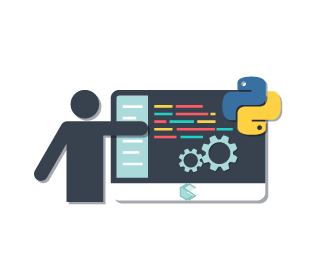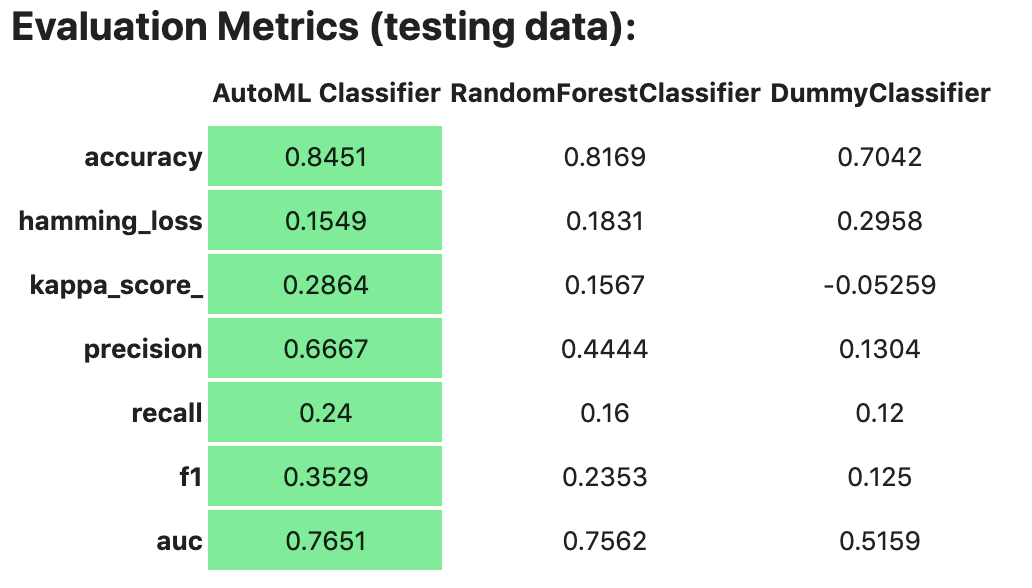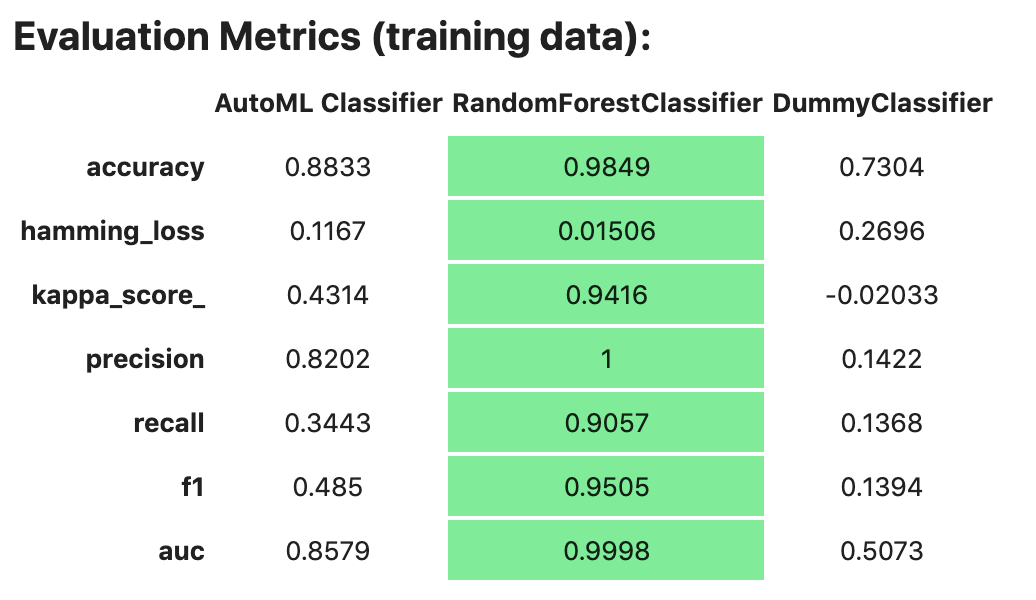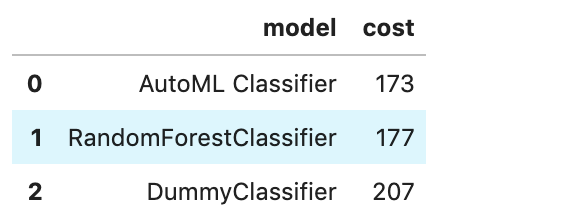# Quick Start

The Accelerated Data Science (ADS) SDK is a Oracle Cloud Infrastructure Data Science and Machine learning SDK that data scientists can use for the entire life cycle of their workflows. You can also use Python methods in ADS to interact with the following Data Science resources:

• Models (saved in the model catalog)

• Notebook Sessions

• Projects

### Install in Your Local Environment

You have various options when installing ADS.

#### Installing the `oracle-ads` base package

```\$ python3 -m pip install oracle-ads
```

#### Installing extras libraries

The `all-optional` module will install all optional dependencies.

```\$ python3 -m pip install oracle-ads[all-optional]
```

To work with gradient boosting models, install the `boosted` module. This module includes XGBoost and LightGBM model classes.

```\$ python3 -m pip install oracle-ads[boosted]
```

For big data use cases using Oracle Big Data Service (BDS), install the `bds` module. It includes the following libraries, ibis-framework[impala], hdfs[kerberos] and sqlalchemy.

```\$ python3 -m pip install oracle-ads[bds]
```

To work with a broad set of data formats (for example, Excel, Avro, etc.) install the `data` module. It includes the fastavro, openpyxl, pandavro, asteval, datefinder, htmllistparse, and sqlalchemy libraries.

```\$ python3 -m pip install oracle-ads[data]
```

To work with geospatial data install the `geo` module. It includes the geopandas and libraries from the viz module.

```\$ python3 -m pip install oracle-ads[geo]
```

Install the `notebook` module to use ADS within the Oracle Cloud Infrastructure Data Science service Notebook Session. This module installs ipywidgets and ipython libraries.

```
```

To work with ONNX-compatible run times and libraries designed to maximize performance and model portability, install the `onnx` module. It includes the following libraries, onnx, onnxruntime, onnxmltools, skl2onnx, xgboost, lightgbm and libraries from the viz module.

```\$ python3 -m pip install oracle-ads[onnx]
```

For infrastructure tasks, install the `opctl` module. It includes the following libraries, oci-cli, docker, conda-pack, nbconvert, nbformat, and inflection.

```\$ python3 -m pip install oracle-ads[opctl]
```

For hyperparameter optimization tasks install the `optuna` module. It includes the optuna and libraries from the viz module.

```\$ python3 -m pip install oracle-ads[optuna]
```

Install the `tensorflow` module to include tensorflow and libraries from the `viz` module.

```\$ python3 -m pip install oracle-ads[tensorflow]
```

For text related tasks, install the `text` module. This will include the wordcloud, spacy libraries.

```\$ python3 -m pip install oracle-ads[text]
```

Install the `torch` module to include pytorch and libraries from the `viz` module.

```\$ python3 -m pip install oracle-ads[torch]
```

Install the `viz` module to include libraries for visualization tasks. Some of the key packages are bokeh, folium, seaborn and related packages.

```\$ python3 -m pip install oracle-ads[viz]
```

Note

Multiple extra dependencies can be installed together. For example:

```\$ python3 -m pip install  oracle-ads[notebook,viz,text]
```

### Getting Started

```import ads
```

Turn debug mode on or off with:

```ads.set_debug_mode(bool)
```

Before you can use ADS for anything involving a dataset (visualization, transformations, or model training), you have to load your data. When ADS opens a dataset, you have the option to provide the name of the column to be the target variable during modeling. The type of this target determines what type of modeling to use (regression, binary, and multinomial classification, or time series forecasting).

There are several ways to turn data into an `ADSDataset`. The simplest way is to use DatasetFactory, which takes as its first argument as a string URI or a `Pandas Dataframe` object. The URI supports many formats, such as Object Storage or S3 files. The class documentation <https://docs.cloud.oracle.com/en-us/iaas/tools/ads-sdk/latest/modules.html>_ describes all classes.

For example:

• From a `Pandas Dataframe` instance:

```import numpy as np
import pandas as pd
df = pd.DataFrame(data.data, columns=data.feature_names)
df["species"] = data.target

# these two are equivalent:
ds = DatasetFactory.open(df, target="species")
# OR
ds = DatasetFactory.from_dataframe(df, target="species")
```

The `ds` (`ADSDataset`) object is `Pandas` like. For example, you can use `ds.head()`. It’s an encapsulation of a Pandas Dataframe with immutability. Any attempt to modify the data yields a new copy-on-write of the `ADSDataset`.

Note

Creating an `ADSDataset` object involves more than simply reading data to memory. ADS also samples the dataset for visualization purposes, computes co-correlation of the columns in the dataset, and performs type discovery on the different columns in the dataset. That is why loading a dataset with `DatasetFactory` can be slower than simply reading the same dataset with `Pandas`. In return, you get the added data visualizations and data*profiling benefits of the `ADSDataset` object.

• Load data from a URL:

```import pandas as pd

```

```import pandas as pd

pd.DataFrame({'c1':[1,2,3], 'target': ['yes', 'no', 'yes']}).to_csv('Users/ysz/data/sample.csv')

ds = DatasetFactory.open('Users/ysz/data/sample.csv',
target = 'target',
type_discovery = False, # turn off ADS type discovery
types = {'target': 'category'}) # specify target type
```

## Data Visualization

ADS offers a smart visualization tool that automatically detects the type of your data columns and offers the best way to plot your data. You can also create custom visualizations with ADS by using your preferred plotting libraries and packages.

To get a quick overview of all the column types and how the column’s values are distributed:

```ds.show_in_notebook()
```

To plot the target’s value distribution:

```ds.target.show_in_notebook()
```

To plot a single column:

```ds.plot("sepal.length").show_in_notebook(figsize=(4,4)) # figsize optional
```

To plot two columns against each other:

```ds.plot(x="sepal.length", y="sepal.width").show_in_notebook()
```

You are not limited to the types of plots that ADS offers. You can also use other plotting libraries. Here’s an example using Seaborn. For more examples, see Data Visualization or the `ads_data_visualizations` notebook example in the notebook session environment.

```import seaborn as sns
sns.set(style="ticks", color_codes=True)
sns.pairplot(df.dropna())
```## Model Training

ADS includes the `OracleAutoMLProvider` class. It is an automated machine learning module that is simple to use, fast to run, and performs comparably with its alternatives. You can also create your own machine learning provider and let ADS take care of the housekeeping.

AutoML provides these features:

• An ideal feature set.

• Minimal sampling size.

• The best algorithm to use (you can also restrict AutoML to your favorite algorithms).

• The best set of algorithm specific hyperparameters.

How to train a model using `ADSDataset`:

```import pandas as pd

# this is the default AutoML provider for regression and classification problem types.
# over time Oracle will introduce other providers for other training tasks.
ml_engine = OracleAutoMLProvider()

# use an example where Pandas opens the dataset
ds = DatasetFactory.open(df, target='variety')

train, test = ds.train_test_split()

automl = AutoML(train, provider=ml_engine)

model, baseline = automl.train(model_list=[
'LogisticRegression',
'LGBMClassifier',
'XGBClassifier',
'RandomForestClassifier'], time_budget=10)
```

At this point, AutoML has built a baseline model. In this case, it is a Zero-R model (majority class is always predicted), along with a tuned model.

You can use `print(model)` to get a model’s parameters and their values:

```print(model)
```
```Framework: automl.models.classification.sklearn.lgbm
Estimator class: LGBMClassifier
Model Parameters: {'boosting_type': 'dart', 'class_weight': None, 'learning_rate': 0.1, 'max_depth': -1, 'min_child_weight': 0.001, 'n_estimators': 100, 'num_leaves': 31, 'reg_alpha': 0, 'reg_lambda': 0}
```

You can get details about a model, such as its selected algorithm, training data size, and initial features using the `show_in_notebook()` method:

```model.show_in_notebook()
```
```Model Name                  AutoML Classifier
Target Variable             variety
Selected Algorithm          LGBMClassifier
Training Dataset Size       (128, 4)
CV                          5
Optimization Metric         recall_macro
Selected Hyperparameters    {'boosting_type': 'dart', 'class_weight': None, 'learning_rate': 0.1, 'max_depth': -1, 'min_child_weight': 0.001, 'n_estimators': 100, 'num_leaves': 31, 'reg_alpha': 0, 'reg_lambda': 0}
Is Regression               None
Initial Number of Features  4
Initial Features            [sepal.length, sepal.width, petal.length, petal.width]
Selected Number of Features 1
Selected Features           [petal.width]
```

From here you have two `ADSModel` objects that can be used in ADS’s evaluation and explanation modules along with any other `ADSModel` instances.

You are not limited to using models that were created using Oracle AutoML. You can promote other models to ADS so that they too can be used in evaluations and explanations.

ADS provides a static method that promotes an estimator-like object to an `ADSModel`.

For example:

```from xgboost import XGBClassifier

...

xgb_classifier = XGBClassifier()
xgb_classifier.fit(train.X, train.y)

```

Optionally, the `from_estimator()` method can provide a list of target classes. If the estimator provides a `classes_` attribute, then this list is not needed.

You can also provide a scalar or iterable of objects implementing transform functions.

## Model Catalog

You can use ADS to save models built with ADS or generic models built outside of ADS to the model catalog. One way to save an `ADSModel` is:

```from os import environ
from joblib import dump
import os.path
import tempfile
tempfilepath = tempfile.mkdtemp()
dump(model, os.path.join(tempfilepath, 'model.onnx'))
model_artifact = prepare_generic_model(tempfilepath)
compartment_id = environ['NB_SESSION_COMPARTMENT_OCID']
project_id = environ["PROJECT_OCID"]

...

mc_model = model_artifact.save(
project_id=project_id,
compartment_id=compartment_id,
display_name="random forest model on iris data",
description="random forest model on iris data",
training_script_path="model_catalog.ipynb",
ignore_pending_changes=False)
```

ADS also provides easy wrappers for the model catalog REST APIs. By constructing a `ModelCatalog` object for a given compartment, you can list the models with the `list_models()` method:

```from ads.catalog.model import ModelCatalog
from os import environ
mc = ModelCatalog(compartment_id=environ['NB_SESSION_COMPARTMENT_OCID'])
model_list = mc.list_models()
```

To load a model from the catalog, the model has to be fetched, extracted, and restored into memory so that it can be manipulated. You must specify a folder where the download would extract the files to:

```import os
path_to_my_loaded_model = os.path.join('/', 'home', 'datascience', 'model')
```

Then construct or reconstruct the `ADSModel` object with:

```from ads.common.model_artifact import ModelArtifact
```

There’s more details to interacting with the model catalog in Model Catalog.

## Model Evaluations and Explanations

### Model Evaluations

ADS can evaluate a set of models by calculating and reporting a variety of task-specific metrics. The set of models must be heterogeneous and be based on the same test set.

The general format for model explanations (ADS or non-ADS models that have been promoted using the `ADSModel.from_estimator` function) is:

```from ads.evaluations.evaluator import ADSEvaluator

evaluator = ADSEvaluator(test, models=[model, baseline], training_data=train)
evaluator.show_in_notebook()
```

If you assign a value to the optional `training_data` method, ADS calculates how the models generalize by comparing the metrics on training with test datasets.

The evaluator has a property `metrics`, which can be used to access all of the calculated data. By default, in a notebook the `evaluator.metrics` outputs a table highlighting for each metric which model scores the best.

```evaluator.metrics
```If you have a binary classification, you can rank models by their calculated cost by using the `calculate_cost()` method.You can also add in your own custom metrics, see the Model Evaluation for more details.

### Model Explanations

ADS provides a module called Machine learning explainability (MLX), which is the process of explaining and interpreting machine learning and deep learning models.

MLX can help machine learning developers to:

• Better understand and interpret the model’s behavior. For example:

• Which features does the model consider important?

• What is the relationship between the feature values and the target predictions?

• Debug and improve the quality of the model. For example:

• Did the model learn something unexpected?

• Does the model generalize or did it learn something specific to the train/validation/test datasets?

• Increase confidence in deploying the model.

MLX can help end users of machine learning algorithms to:

• Understand why the model has made a certain prediction. For example: - Why was my bank loan denied?

Some useful terms for MLX:

• Explainability: The ability to explain the reasons behind a machine learning model’s prediction.

• Global Explanations: Understand the behavior of a machine learning model as a whole.

• Interpretability: The level at which a human can understand the explanation.

• Local Explanations: Understand why the machine learning model made a single prediction.

• Model-Agnostic Explanations: Explanations treat the machine learning model (and feature pre-processing) as a black-box, instead of using properties from the model to guide the explanation.

MLX provides interpretable model-agnostic local and global explanations.

How to get global explanations:

```from ads.explanations.explainer import ADSExplainer

# our model explainer class

# let's created a global explainer
global_explainer = explainer.global_explanation(provider=MLXGlobalExplainer())

# Generate the global feature importance explanation
importances = global_explainer.compute_feature_importance()
```

Visualize the top six features in a bar chart (the default).

```# Visualize the top 6 features as a bar chart
importances.show_in_notebook(n_features=6)
```

Visualize the top five features in a detailed scatter plot:

```# Visualize a detailed scatter plot
importances.show_in_notebook(n_features=5, mode='detailed')
```

Get the dictionary object that is used to generate the visualizations so that you can create your own:

```# Get the dictionary object used to generate the visualizations
importances.get_global_explanation()
```

MLX can also do much more. For example, Partial Dependence Plots (PDP) and Individual Conditional Expectation explanations along with local explanations can provide insights into why a machine learning model made a specific prediction.

For more detailed examples and a thorough overview of MLX, see the MLX documentation.